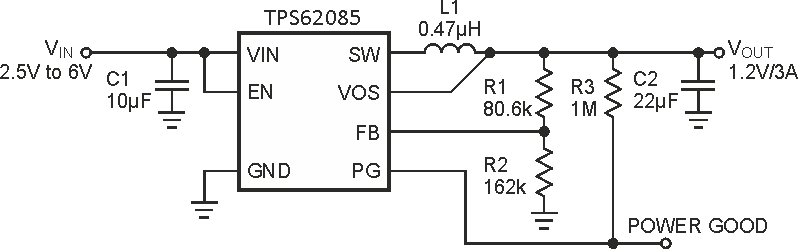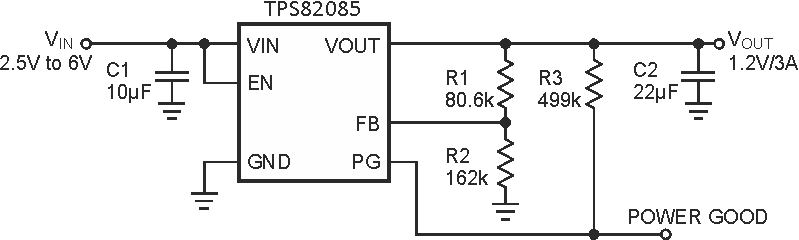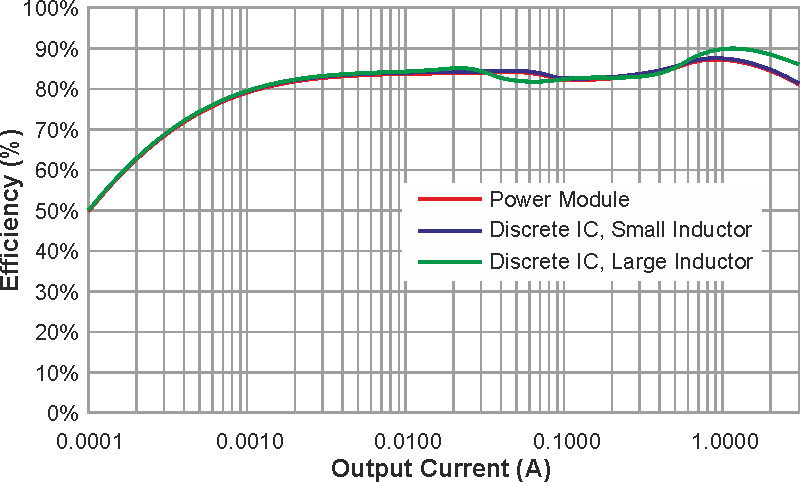# Power Module or Discrete Power Solution: What's Best for Your Design?

Chris Glaser, Texas Instruments

Electronic Design

Small solution sizes and high integration levels are required for extremely space-constrained systems, such as optical modules and solid-state drives (SSDs). While integrated power modules tend to be highly desired in these and other small-size applications, they were previously limited to lower-load-current applications, with discrete power supplies used elsewhere. However, the latest power modules, which now deliver 3 A, allow for much smaller solution sizes while giving acceptable performance. In this article, I compare the efficiency, solution size, and cost of a power module to an equivalent discrete solution.

 а)b)Figure 1. Typical schematics of both a discrete power supply (a) and power
module (b) for a 1.2-V output voltage are very simple.

Figure 1 shows the typical schematic for an example step-down converter, which is available as either a discrete integrated circuit (IC) or MicroSiP1 power module. Both solutions deliver 3 A of output current to this example’s 1.2-V rail. The passive components required are identical for each device except for the inductor, which is integrated into the module. Both devices use the DCS-Control topology2, which gives excellent transient response and good output-voltage regulation; both are required in communications equipment and storage applications.

## Efficiency

When evaluating the efficiency of an integrated MOSFET step-down converter, you must use the same inductor to get an accurate comparison between two different devices. Because the vast majority of losses occur in either the IC or inductor, and since the IC’s loss characteristics are unchangeable, the inductor is the only knob with which to tune the efficiency. By their nature, power modules incorporate a smaller inductor, which has a higher dc resistance (DCR), to minimize their total size. Typically, a discrete power supply uses a larger inductor, which has a lower DCR, to maximize efficiency. There’s a clear tradeoff between size and efficiency.

Figure 2 shows the efficiency of the discrete power supply and power module with the inductor used in their respective datasheet’s measurements. A larger inductor is used for the discrete IC and a smaller inductor for the power module. A third curve shows the efficiency of the discrete power supply with the same, smaller inductor incorporated in the power module.Figure 2. The efficiency of a discrete power supply (TPS62085) and power module (TPS82085) for a 1.2-V output voltage is nearly identical until the arrival of heavier load currents.

As expected, the discrete power supply with the smaller inductor gives the same efficiency as the power module. The efficiency is essentially the same between all three options until higher load currents. At these currents, the DCR difference of the inductors is most significant and responsible for the efficiency change.

## Solution Size

The main advantage of choosing a power module is smaller solution size. A MicroSiP module stacks components in the z-direction, which makes the smallest x-y area possible. However, stacking components also increases the height of the solution. The lowest height solution is found with a discrete power supply.

Table 1. A discrete power supply (TPS62085) may achieve a lower
height than a power module (TPS82085), although the power
module always has the smallest footprint.
 Solution size (mm2) Maximum solution height (mm) Power module 35 1.33 Discrete IC small inductor 45 1 Discrete IC large inductor 62 1.6

Table 1 summarizes the solution size and solution height based on the evaluation module (EVM) for each device. The power module saves 22% in board area but increases the height by 33% compared to an equivalent discrete power supply. You can save 43% in board area when using the power module instead of the larger inductor for the discrete power supply.

## Cost

With all other items being equal, a power module costs more than a discrete power-supply IC because of the integrated inductor and its component and assembly cost. However, the cost of a complete power supply extends beyond the cost of just the power-supply IC. Other expenses include the cost to pick and place each individual component onto the printed circuit board (PCB) during assembly; the cost to qualify, order, and stock every item in the bill of materials (BOM); the cost and risk of PCB layout (because routing the inductor and switch node [SW pin] requires careful thought and adds some risk); and the PCB cost itself – larger PCBs are more expensive. A power module is priced higher but saves PCB space and simplifies the design effort.

Table 2. Summary of tradeoffs between a discrete power supply (TPS62085)
and power module (TPS82085).
 Efficiency at 1-A load Efficiency at 3-A load Solution size (mm1) Maximum solution height (mm) Component count Device cost (1 ku) Solution cost (1 ku) Power module 87% 81% 35 1.33 5 \$2.75 \$2.86 Discrete IC small inductor 87% 81% 45 1 6 \$0.95 \$1.36 Discrete IC large inductor 90% 86% 62 1.6 6 \$0.95 \$1.36

Table 2 summarizes the tradeoffs between a module and a discrete power-supply IC. For the total solution cost, the inductor cost is estimated at \$0.30, the capacitors at \$0.05 each and the resistors at \$0.005 each.

## Conclusion

New 3-A power modules occupy 22% less space than an equivalent discrete IC implementation while maintaining the same efficiency. However, the maximum height increases, as does the solution cost. This added cost is partially offset by fewer components on the PCB and in the BOM, and by faster and easier PCB layout.

A power module offers a validated and specified solution, while a discrete power supply enables more customization to the application. Both the power module and discrete power-supply IC are valid solutions with various tradeoffs for space-limited applications.

## Materials on the topic

electronicdesign.com